# Algebra II : Real Numbers

## Example Questions

1 3 Next →

### Example Question #21 : Number Theory

Which of the following are NOT real numbers?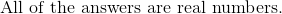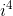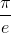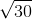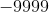Explanation:

Real numbers may include positive and negative integers, rational numbers, and also irrational numbers.

Rational numbers are terms that can be expressed as ratios of numbers, such as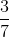.

Real numbers cannot include infinity or imaginary numbers, such as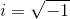.

However, the imaginary term provided in the answer choice is not fully simplified.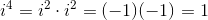This value is a real number.

The termis an irrational number, and is considered a real number.

The termis also irrational, and is a real number.

The correct answer is:1 3 Next →

### All Algebra II Resources